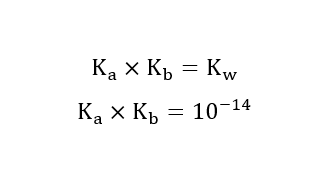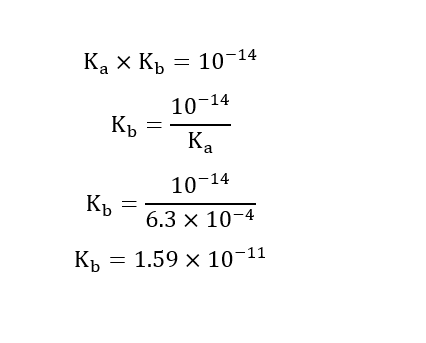# Given that KaKa for HFHF is 6.3×10−46.3×10−4 at 25 °C,25 °C, what is the value of KbKb for F−F− at 25 °C?25 °C?Kb= Given that KbKb for (CH3)3N(CH3)3N is 6.3×10−56.3×10−5 at 25 °C,25 °C, what is the value of KaKa for (CH3)3NH+(CH3)3NH+ at 25 °C?25 °C?Ka=

Question
12 views
Given that KaKa for HFHF is 6.3×10−46.3×10−4 at 25 °C,25 °C, what is the value of KbKb for F−F− at 25 °C?25 °C?
Kb=

Given that KbKb for (CH3)3N(CH3)3N is 6.3×10−56.3×10−5 at 25 °C,25 °C, what is the value of KaKa for (CH3)3NH+(CH3)3NH+ at 25 °C?25 °C?
Ka=
check_circle

Step 1

The relationship between Ka and kb is,Step 2

Given:

Ka for HF is 6.3×10−4

Calculation for Kb of  F-:...

### Want to see the full answer?

See Solution

#### Want to see this answer and more?

Solutions are written by subject experts who are available 24/7. Questions are typically answered within 1 hour.*

See Solution
*Response times may vary by subject and question.
Tagged in